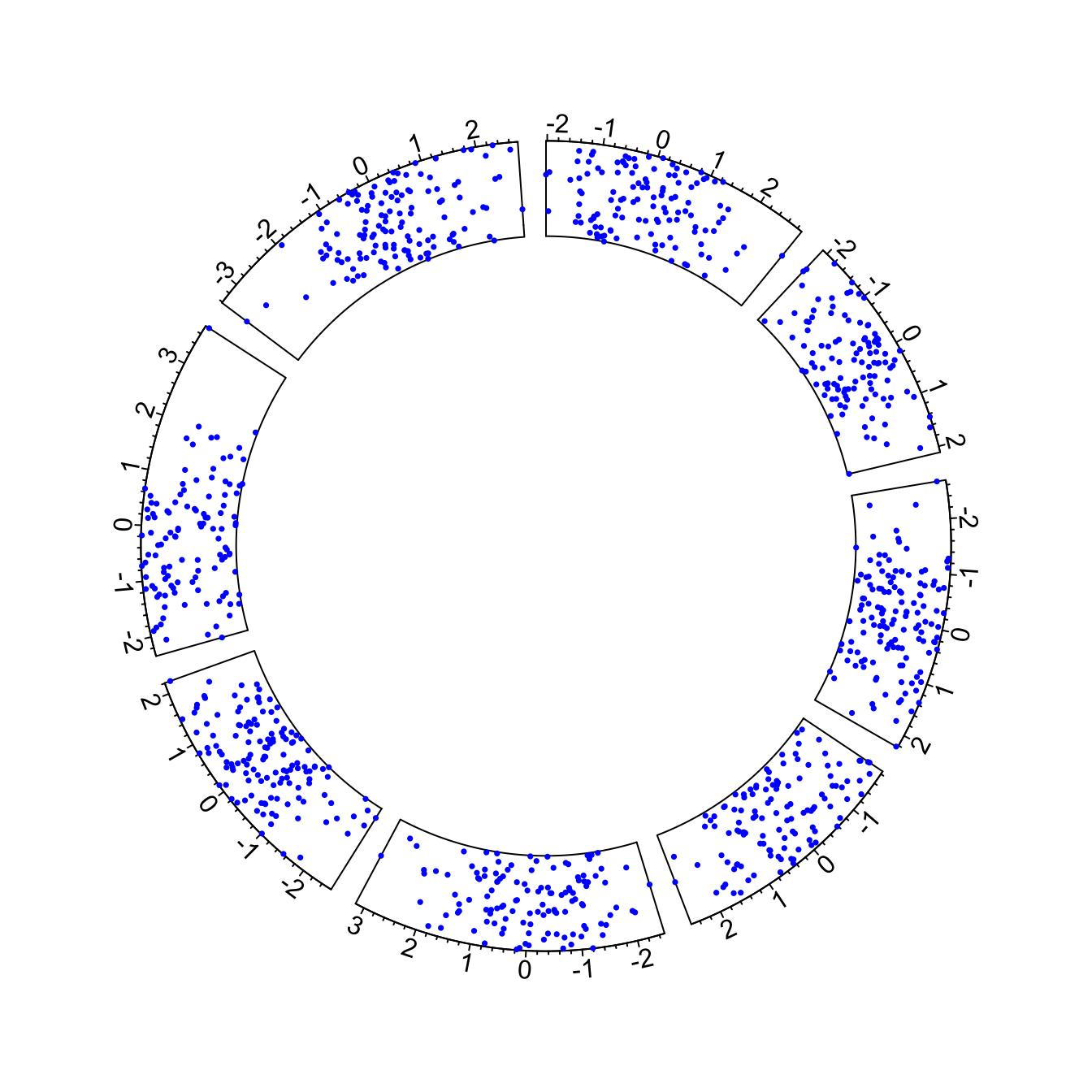# Introduction to the circlize package

This post is an introduction to the `circlize` package: the ultimate way to build circular charts with R. It shows how to initialize a circular section and fill it with a scatterplot.

In R, circular plots are made using the `circlize` package. Circular plots are composed by several regions (8 here), each representing a level of a factor. Three steps are required to build a circular plot:

• Step 1: Initialize the chart with `circos.initialize()`. Provide the factor vector, and the numeric values to use for the X axis. The circle will be split in as many zone as the number of levels present in your factor. Each region will be as long as the coresponding x axis.

• Step2: Build the regions with `circos.trackPlotRegion()`. You have to specify the factors once again, and tell what to use for the Y axis if needed.

• Step3: Add a chart in each region. Here `circos.trackPoints()` is used to build a scatterplot. See chart #226 for other chart types.``````# Upload library
library(circlize)

# Create data
data = data.frame(
factor = sample(letters[1:8], 1000, replace = TRUE),
x = rnorm(1000),
y = runif(1000)
)

# Step1: Initialise the chart giving factor and x-axis.
circos.initialize( factors=data\$factor, x=data\$x )

# Step 2: Build the regions.
circos.trackPlotRegion(factors = data\$factor, y = data\$y, panel.fun = function(x, y) {
circos.axis()
})